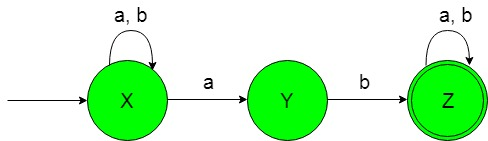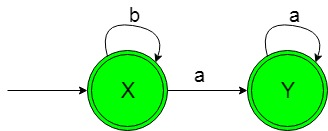# Designing Non-Deterministic Finite Automata (Set 5)

• Difficulty Level : Basic
• Last Updated : 20 Nov, 2019

Prerequisite: Finite Automata Introduction
In this article, we will see some designing of Non-Deterministic Finite Automata (NFA).

Problem-1: Construction of a minimal NFA accepting a set of strings over {a, b} in which each string of the language contain ‘ab’ as the substring.
Explanation: The desired language will be like:

`L1 = {ab, abba, abaa, ...........}`

Here as we can see that each string of the above language contains ‘ab’ as the substring but the below language is not accepted by this NFA because some of the string of below language does not contain ‘ab’ as the substring.

`L2 = {bb, b, bbbb, .............}`

The state transition diagram of the desired language will be like below:In the above NFA, the initial state ‘X’ on getting ‘a’ as the input it either remains in the state of itself or transit to a state ‘Y’ and on getting ‘b’ as the input it remains in the state of itself.

The state ‘Y’ on getting ‘b’ as the input it transmits to a final state ‘Z’. The final state ‘Z’ on getting either ‘a’ or ‘b’ as the input it remains in the state of itself.

Problem-2: Construction of a minimal NFA accepting a set of strings over {a, b} in which each string of the language is not containing ‘ab’ as the substring.
Explanation: The desired language will be like:

`L1 = {b, bb, bbbb, ...........}`

Here as we can see that each string of the above language is not containing ‘ab’ as the substring But the below language is not accepted by this NFA because some of the string of below language is containing ‘ab’ as the substring.

`L2 = {ab, aba, ababaab..............}`

The state transition diagram of the desired language will be like below:In the above NFA, the initial and final state ‘X’ on getting ‘b’ as the input it remains in the state of itself and on getting ‘a’ as the input it transits to another final state ‘Y’.

Another final state ‘Y’ on getting ‘a’ as the input it remains in the state of itself.

My Personal Notes arrow_drop_up